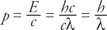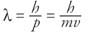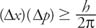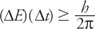## Quantum Mechanics

Not only was classical mechanics unsuccessful in explaining motions near the speed of light, it also could not explain the behavior of matter on the atomic level. Quantum mechanics is required to analyze the behavior of molecules, atoms, and nuclei.

A blackbody is an ideal thermal object that absorbs all radiation falling on it at low temperatures and is also a perfect radiator. The curves of radiation intensity versus wavelength could not be explained by classical physics. Max Planck (1858–1947) developed an equation for blackbody radiation that agreed with the data. This derivation required two assumptions:

• The vibrating molecules emitting the radiation could have only certain discrete amounts of energy, given by E n = nhf, where n is called a quantum number, F is the frequency, and h is Planck's constant given by h = 6.626 × 10 −34 joule‐seconds.
• Molecules emit energy in units called quanta, now called photons. They do this by jumping from one energy state to another. The energy of the light quanta emitted by the jump between energy states is given by E = hf, or in terms of wavelength,The radical nature of Planck's vision is the assumption of quantized energy states. The terms discrete and quantum referred to considering the energy as coming in packets instead of as a continuous flow; thus, the molecule will change energy states only if the amount of energy absorbed or radiated is a discrete amount of energy.

## Photoelectric effect

The photoelectric effect is the emission of electrons from certain metals when light shines on the metallic surface. The emitted electrons are called photoelectrons. A number of aspects of the effect were puzzling:

• For a given metal, the light has to be of at least a minimum frequency, called the cutoff frequency or the threshold frequency.
• The kinetic energy of the photoelectrons is not dependent upon the intensity of the light causing the photoelectric effect.
• The maximum kinetic energy of the photoelectrons increases with increasing light frequency.
• Electrons are emitted almost immediately from the surface; that is, no build‐up of energy flowing into the molecules is necessary. Figure shows a sketch of the maximum kinetic energy versus the frequency of light incident upon the metal.Figure 1 The photoelectric effect.

Einstein explained the photoelectric effect, using Planck's quantum hypothesis and the conservation of energy. His equation is K.E. max = hfQ, where Q is called the work function. The work function is the energy required to release the electron from a particular metal. The energy of the incoming photons, or quanta, is hf; therefore, the photoelectric equation simply states that the energy of the ejected electrons is the difference between the energy absorbed from the quanta of light and the energy required to escape from the material.

The unexplained observations described above can be illuminated by the following arguments.

• A certain threshold frequency of the incident light is necessary to provide a quanta of sufficient energy to eject an electron.
• As the intensity of the incident light increases, so will the number of emitted electrons, but their kinetic energies will not increase.
• As the frequency increases, so does the energy of the photons so that the ejected electrons will have greater kinetic energy.
• A low‐light intensity indicates few quanta, but if those are of sufficient energy, some electrons will be emitted immediately.

## Compton scattering

Additional evidence for the quantized nature of electromagnetic waves came from the Compton effect, named for Arthur Compton (1892–1962). Compton scattering involves the scattering of a high‐energy, e‐ray photon and an electron. The scattered photon has less energy than the original photon, which can be seen as a change in wavelength. Compton explained this by assuming that the photon behaves like a particle when interacting with the electron. The conservation of momentum and energy used for elastic collisions of billiard balls could mathematically explain the experimental observations. The scattering effect is dependent upon the angle but not the wavelength. The small shift in wavelength would be too difficult to detect with less energetic photons, such as light photons.

## Particle-wave duality

The photoelectric effect and the Compton effect again point to the duality of the nature of electromagnetic radiation. The models of light as a wave and also as a particle complement each other. When the photons of electromagnetic radiation are of relatively high energy, the wavelengths are short. Then the photon acts more like a particle than a wave. For example, the Compton photons were high‐energy x‐rays. When the photons of electromagnetic radiation have relatively low energy, the wavelengths are long. Radio waves are an example of less energetic photons that act more like waves than particles.

## De Broglie waves

Louis de Broglie (1892–1987) postulated that because photons have both wave and particle characteristics, perhaps particles also have wave characteristics. From the energy of the photon,the momentum of a photon can be derived:De Broglie hypothesized that material particles with momentum p should have a wave nature and a corresponding wavelength given by his equation:Note that the de Broglie wavelength is directly proportional to h, which is a constant to the –34 power. With the relatively large masses and velocities of ordinary life, the de Broglie wavelengths are so small that they are virtually undetectable.

## The Heisenberg uncertainty principle

The Heisenberg uncertainty principle, formulated by Werner Heisenberg (1901–1976), states that it is impossible to simultaneously measure a particle's position and velocity exactly. Specifically, the uncertainty in the measurements are given byAnother form of the expression refers to the uncertainty in measurements of energy and timeIn principle, it is possible to make exact measurements in classical physics; however, even in principle making exact measurements is not possible in quantum mechanics. Consider finding the exact position of a charged particle that produces a spot of light when hitting a phosphor. The exact position is known, but information about the particle's momentum has changed. Or consider viewing an object under a microscope. In order to see the object, some photons must reflect off it to the eye of a viewer. These incident photons will cause uncertainties in the measurement. In other words, the very act of the measurement procedure in quantum mechanics introduces uncertainty into the data collected.